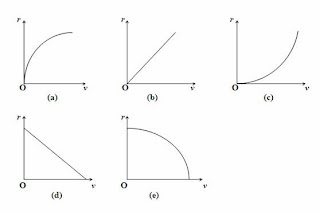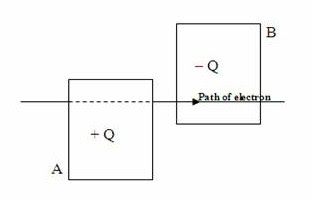## Pages

`“Life is like riding a bicycle.  To keep your balance you must keep moving.”–Albert Einstein`

## Friday, February 24, 2012

### AP Physics B & C - Multiple Choice Practice Questions on Magnetic Fields

“The best way to find yourself is to lose yourself in the service of others."
– Mahatma Gandhi

Questions on magnetic fields due to current carrying wires and the magnetic force on moving charges and current carrying wires are generally interesting. Such questions have been discussed on many occasions on this site. You can access them by clicking on the label ‘magnetic field’ below this post. Note that the percentage goal from this section for AP Physics C Electricity and Magnetism Exam is 20% while that for AP Physics B Exam is 4% (for the May 2012 Exam)
Today we will discuss a few more multiple choice practice questions in this section
(1) An electron moves with speed v in a direction perpendicular to a constant magnetic field B. Which one of the following graphs best represents the radius r of the path of the electron as a function of its speedThe radius r of the circular path followed by the electron in the perpendicular magnetic field B is given by
r = mv/qB where m is the mass of the electron and q is its charge.
[The above expression is obtained by equating the centripetal force to the magnetic force on the electron:
mv2/r = qvB]
In a constant magnetic field the radius r is directly proportional to the speed v and the graph shown in option (b) is the answer.(2) Two vertical metal plates A and B are arranged in an evacuated chamber with their planes parallel to each other and they carry charges + Q and – Q as indicated in the adjoining figure. A uniform magnetic field B exists in the region between the plates. An electron entering with horizontal velocity ‘v’ rightwards in the central region between the plates is found to proceed undeviated throughout the region. What is the direction of the magnetic field? (Ignore the effect of gravity)
(a) horizontally leftwards
(b) horizontally towards plate A
(c) horizontally towards plate B
(d) vertically downwards
(e) vertically upwards
Since the electron is negatively charged, the electric field in the region between the plates will exert a horizontal force on the electron. This force is directed towards the positive plate A. Since the electron proceeds undeviated throughout the region between the plates, the magnetic force on the electron must be equal and opposite to the electric force. This means that the magnetic force on the electron is directed horizontally towards the plate B
The rightward moving electron is equivalent to a leftward current. Therefore, on applying Fleming’s left hand rule, we find that the magnetic field must act vertically upwards.
[To apply Fleming’s left hand rule, hold the fore finger, middle finger and thumb of your left hand along mutually perpendicular directions such that the middle finger points along the direction of the current (horizontally leftwards in the present case) and the thumb points along the direction of the force (horizontally towards plate B in the present case). Your fore finger will then point vertically upwards].
(3) If the magnitude of the electric field in the above question is E and the mass and speed of the electron are respectively m and v, what is the magnitude of the magnetic field in between the plates?
(a) E/v
(b) Ev/m
(c) E/mv
(d) v/E
(e) E
Since the electron proceeds undeviated under the action of crossed electric and magnetic fields, we can equate the magnitudes of the magnetic and electric forces. Therefore, we have
Bev = Ee
This gives B = E/v.
[The mass of the electron given in the question just serves the purpose of a distraction]

(4) A charged particle entering at right angles to a uniform magnetic field has its trajectory as shown in the figure, during a small time interval. The magnetic field is directed normally into the plane of the trajectory and the speed of the particle is decreasing continuously. Which one among the following statements is correct?
(a) The particle is negatively charged and is moving in the clockwise sense
(b) The particle is negatively charged and is moving in the anticlockwise sense
(c) The particle is positively charged and is moving in the clockwise sense
(d) The particle is positively charged and is moving in the clockwise sense
(e) Nothing can be conclusively mentioned about the motion of the particle since the nature of its charge is not given
The particle must be moving in the clockwise sense since the radius of its initial path has to be greater because of the greater initial speed.
[We have radius r = mv/qB so that r α v. Qualitatively speaking, a particle with larger velocity cannot be easily affected by a force].
On applying Fleming’s left hand rule (motor rule) it is seen that the particle is negatively charged.
[When you hold the fore finger of your left hand along the direction of the magnetic field (normally into the plane of the figure) and the middle finger along the initial direction of the particle, you will find that the thumb is pointing radially outwards and not inwards as required for the curved trajectory given in the figure. This indicates that the direction of the conventional current is opposite to the direction of motion of the particle. Therefore the particle must be negatively charged.
(5) A straight wire of length 0.2 m is oriented along the z-axis. It carries a current of 5 A flowing along the positive z-direction. The magnetic force acting on this wire when it is placed in a magnetic field of flux density 0.4 j – 0.5 k tesla is (i, j and k are unit vectors along the x, y and z-directions respectively)
(a) – 0.4 j N
(b) 0.4 k N
(c) 0.9 i N
(d) – 0.4 i N
(e) 0.9 i N
The magnetic force F on the wire is given by
F = I L×B = 5[(0.2 k)×(0.4 j – 0.5 k)] = – 0.4 i newton.

[The magnetic force on the wire acts along the negative x-direction].